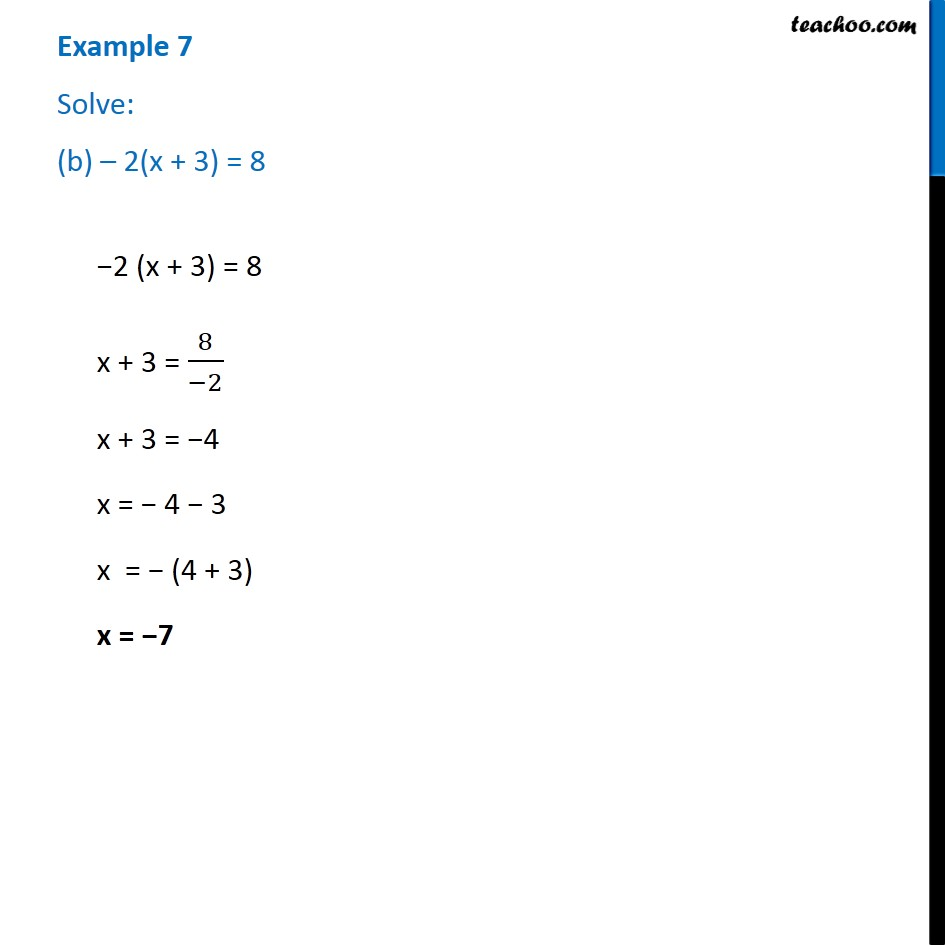1. Chapter 4 Class 7 Simple Equations
2. Serial order wise
3. Examples

Transcript

Example 7 Solve: (b) – 2(x + 3) = 8−2 (x + 3) = 8 x + 3 = 8/(−2) x + 3 = −4 x = − 4 − 3 x = − (4 + 3) x = −7